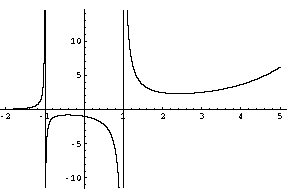Name: Sam Who is asking: Student Level of the question: Secondary Question: Hi, i am trying to find the point of inflexion for the curve y = ex/(x2-1) and i got a really complex expression for y". I can't seem to solve x4-4x3+4x2+4x+3=0 so does that mean there is no point of inflexion? Hi Sam, I got the same second derivative as you. I also don't see a solution to x4-4x3+4x2+4x+3 = 0 and I don't know a nice way to show that is has no solutions. I used Mathematica to sketch a graph of y = ex/(x2-1) for x between -2 and 5 and I gotGraph of y = ex/(x2-1) The curve changes concavity at x = -1 and x = 1 but I don't believe there are any inflexion points. Then I had Mathematica solve x4-4x3+4x2+4x+3 = 0 and it gave me four solutions, none of which are real so your conclusion is correct, there are no inflexion points. Penny